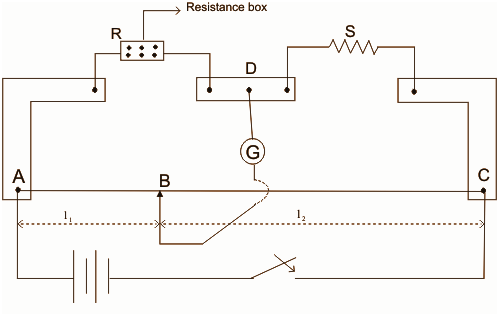# Meter Bridge

A meter bridge also called a slide wire bridge is an instrument that works on the principle of a Wheatstone bridge. A meter bridge is used in finding the unknown resistance of a conductor as that of in a Wheatstone bridge.

## What Is Wheatstone Bridge?

A Wheatstone bridge is a kind of electrical circuit used in measuring an electrical resistance, which is unknown by balancing its two legs of the bridge circuit, where one of the legs includes an unknown component. Samuel Hunter Christie created this instrument in the year 1833 and was improved and also simplified by Sir Charles Wheatstone in the year 1843. The digital multimeters in today’s world provide the simplest forms in measuring the resistance. The Wheatstone Bridge can still be used in measuring light values of resistances around the range of milli-Ohms.

### How Is A Meter Bridge Used In Finding The Unknown Resistance?

A meter bridge is an apparatus utilized in finding the unknown resistance of a coil. The below figure 12 is the diagram of a useful meter bridge instrument.In the above figure, R is called as the Resistance, P is the Resistance coming across AB, S is the Unknown Resistance, Q is the Resistance between the joints BD.

AC is the long wire measuring 1m in length and it is made of constantan or manganin having a uniform area of the cross-section Such that L1 + L2 = 100

Assuming that L1 = L => L2 = 100 – L

Relation obtains the unknown resistance ‘X’ of the given wire:
X = RL2/L1 = R(100 – L)/L

And the specific resistance of the material for a given wire is obtained by the relation = (3.14) r2X/l

where, r = the radius of the cable and also l = length of the wire.

The devices required in finding the unknown resistance of a conductor using a meter bridge are:

• Meter bridge
• Resistance box
• Galvanometer
• Unknown Resistance of a length 1 m
• Screw gauge
• Connecting Wires
• Jockey
• One way key

In the meter bridge, one of the lateral kinds of resistances is replaced by a wire having a length of the uniform cross section of about 1m. The other pair consists of one known and an unknown pair of resistances. The one part of the galvanometer is connected in between both resistances, whereas the other part of the wire is finding the null point where the galvanometer is not showing any deflection. At this point, the bridge is said to be balanced.

### Procedure For Finding The Unknown Resistance Using Meter Bridge

• Collect the instruments and prepare connections as shown in the above figure.
• Take some suitable kind of resistance ‘R’ from the resistance box.
• Touch jockey at the point A; look that there exists a deflection in galvanometer on one of the sides, then contact the jockey on point C of wire, then the deflection in galvanometer has to be on another side.
• Find the position of the null point having deflection in the galvanometer that becomes zero. Note the length AB (l) BC = (100 – l).
• Continue the above method for some different values of the ‘R’. Note at least some 5 readings.
• Consider the point where galvanometer shows a 0 deflection; this is called balance point.
• Now, Measure the length of given wire by the use of ordinary scale and radius of the wire by the utilization of a screw gauge, (Take at least five readings).
• Calculate Mean Resistance of Single Unknown Resistance = Total Sum of resistances of Unknown resistance from the above five readings)/5.